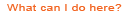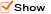# Mathematics Classroom Context: Coursetaking in Mathematics|
• Use the tabs to view student performance by gender, race/ethnicity, and grade level.
• Use the drop down menu when viewing student group performance to see data for different assessment years.
• See the data displayed in other chart formats by clicking on the "chart options" button below each figure.
• Click on the link to the NAEP Data Explorer under each table to conduct a significance test, which is used to determine whether the changes or differences between two result numbers are statistically significant

As part of the 2012 long-term trend assessment, 13-year-old students were asked about the mathematics courses they were taking this year. The full text of the question is below.

What kind of mathematics are you taking this year?

A. I am not taking mathematics this year
B. Regular mathematics
C. Pre-algebra
D. Algebra
E. Other

As part of the 2012 long-term trend assessment, 17-year-old students were asked about the mathematics courses they have taken. The full text of the question is below.

Counting what you are taking now, have you ever taken any of the following mathematics courses?

A. General, business, or consumer mathematics
B. Pre-algebra or introduction to algebra
C. First-year algebra
D. Second-year algebra
E. Geometry
F. Trigonometry
G. Pre-calculus or calculus